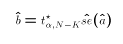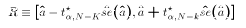## G. Statistical Inference

#### Confidence Intervals

We will define confidence intervals for a population parameterin the following situation:
1. We have a sample of size N
2. We already have an estimatorthat we know is unbiased
3. We also know from some other results thatThe value of k is going to depend on the situation in which we are estimating.
A confidence interval for a parameter, given and estimatoris a rangecomputed from the sample data so that the true valuelies inin a pre-determined (before sampling) percentage of all possible samples from the population.
The pre-determined percentage is called the confidence level. It is usually denoted by "1-." whereis the significance level.
Note that the range is defined by a pair of numbersandwhich by defintion are functions of a sample, so they are random variables.
The definition now says thatmust be chosen so that the true valuemust lie in the range in 1-of the samples. In other words:orThe only random (sample-dependent) values in (*) areand. This explains why we need to know the sampling distribution of, otherwise we could not determine a value ofthat makes (*) true.
We started with the presumption that left hand side of the inequality in (*) equals the absolute value of the numerator of adistribution. Dividing both sides of the inequality by the denominator allows us to write (*) asUsing tables of the t-distribution, we find the critical valuethat makes this inequality true. In other words, set:whereThis leads to the much more familiar expression for a confidence interval for:### 3 Steps to construct a Confidence Interval

1. Choose your confidence level 1-, determine the value of k for your situation (discussed later), and look up the value of.
2. Compute the estimateand its estimated standard error.
3. Computeand finally.

#### Hypothesis Testing

A hypothesis test solves the following problem:
Make a decision to either REJECT or FAIL-TO-REJECT a hypothesis that a population parameter equals a particular value. Do this while setting the proportion of samples in which rejection occurs when the hypothesis is true to a pre-determined value.
The proportion of samples in which FALSE rejection occurs is called the level of significance of the test, usually denoted. We also call this the probability of a Type I error. A Type II error is failing to reject the hypothesis when it is actually false.

When constructing a confidence interval, the interval depends on the sample and so it is random. When conducting a hypothesis test, the decision (reject or fail-to-reject) depends on the sample and so the decision made is random before the sample is drawn.

### 5 Required Elements of a Hypothesis Test

1. The NULL Hypothesis, which states the value to test.
2. The ALTERNATIVE Hypothesis, which are the values to test against
3. The probability of Type I error, usually called ALPHA (), also known as the test's level of significance. The value ofis chosen by the researcher!
4. A test statistic which:
1. can be computed with sample information
2. whose distribution is known when the NULL hypothesis is true
5. The values of the test statistic for which the NULL is rejected in favour of the ALTERNATIVE. This is sometimes called the CRITICAL REGION of the test.
Each of these required elements can be determined before anything is done with the sample information. Once each of these elements is specified correctly, carrying out a hypothesis test is simple.

### Two Ways to do a Hypothesis Test: Use Critical Region or p-value

1. Way 1: Check if Calculated Test Statistic falls in Critical Region or not
To illustrate the two ways to actually make the decision to reject or fail to reject H0, we'll take the common example of a two-sided Z test. That is, part 4 of the 5 required elements in this case is a test statistic that follows the standard normal distribution when the null hypothesis is in fact true. Let's say you are willing to take a one-in-ten chance that you reject H0 when in fact it is true. That is,= .10. If you look up values of the z distribution you will find that z*(.10)=1.645 in this case. (You can also have Stata calculate this for you by typing di invnorm(.95) (yes, .95 not .90 because this example is a two-sided test). So part five of the five required elements would be:
Example Crticical Region:  Reject H0 if |zcalc| > 1.645.

Given your data, you calculate the test statistic and find it equals 0.731. Therefore, you could complete this test in the following way: The value of the test statistic in the sample is .731. Since |.731| is < 1.645, I fail to reject the null hypothesis. . Here is a picture that goes along with this decision.2. Way 2: Check whether p-value for the calculated test statistic is greater or less than your chosen level of significance ().
What is a p-value? The p-value of a calculated test statistic is the probability under H0 that the test statistic takes on a value greater in absolute value than the calculated value. (For one-sided test the appropriate p-value would be the probability that the test statistic takes on a value either greater or less than the calculated test statistic but not both. Stata usually reports p-values associated with two-sided tests when they are possible.) Why is the p-value useful? Let's return to the example to see why. With zcalc=.731 and alpha=.1 we fail to reject the two-sided hypothesis because .731 is not in the critical region z > |1.645|. Suppose we knew Prob(|Z|>.731). That is, suppose we knew the area shaded blue in the figure below:In fact, one can look up the area of the shaded region using Z tables, or you can let Stata do the work by typing di 2*normprob(-.731). Either way, you find that the area of the region equals .465. That is, there is a .465 chance that a Z random variable will take on a value greater in absolute value than .731. This would be the p-value of the calculated test statistic.

Notice that .465 is greater than alpha = .1, which is the area corresponding to P(|Z|>1.645).

Suppose Stata tells you the p-value of a calculate test statistic. Looking at the figure above you should be able to see that you can make the decision about the hypothesis test without checking whether the calculated value lands in the critical region or not. If the p-value is greater than, then we know that we should fail to reject H0. On the other hand, if the p-value is less thanthen this means the calculated test statistic does lie in the critical region and we should reject H0.

Why use the p-value method rather than the critical region method? As long as the statistical package makes it easy to get the p-value then you don't have to look up the critical value of the test statistic as well, which saves you time. Why doesn't a statistical package like Stata provide critical values as well as p-values? Because critical values depend upon your own personal choice ofwhich the program doesn't know ahead of time. The p-value depends only upon H0, the calculated value of the test statistic (which comes from the sample), and whether the test is one-sided or two-sided. Can using p-values be confusing? Yes!. Notice that the inequality switches when using the p-value approach than when using the critical region approach. That is, the decision rule Reject H0 if calculated test statistic is greater in absolute value than the critical value becomes Reject H0 if p-value is less than. If you mix this up you will come to exactly the wrong conclusion! Also, you must avoid comparing apples and oranges. Whichever decision rule you choose to apply, always compare test statistics to critical values and p-values to significance levels.

### 3 Steps to carry out a Hypothesis Test

1. Specify the 5 required elements of a Hypothesis test listed above.
2. Using the sample data, compute either the value of the test statistic or the p-value associated with the calculated test statistic.
3. Use one of two possible decision rules (don't mix them up!):
1. Reject the NULL hypothesis if the test statistic falls in the critical region. Fail to reject the NULL if the test statistic does not fall in the critical region.
2. Reject the NULL hypothesis if the p-value is less that. Fail to reject the NULL if the p-value is greater than.

This document was created using HTX, a (HTML/TeX) interlacing program written by Chris Ferrall. Document Last revised: 1997/1/5

## End of Document glossary

[glossary Contents] [Previous File]